# Diamond perimeter

Calculate the diamond circumference which area is 288 cm square and one diagonal has a size of 124 cm.

Result

x =  248.174 cm

#### Solution:Leave us a comment of example and its solution (i.e. if it is still somewhat unclear...):

Showing 0 comments:Be the first to comment!#### To solve this example are needed these knowledge from mathematics:

Pythagorean theorem is the base for the right triangle calculator.

## Next similar examples:

1. Diagonals of diamondFind the area and circumference of the diamond ABCD with 15m and 11m diagonals.
2. Acreage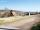Plot has a diamond shape, its side is 25.6 m long and the distance of the opposite sides is 22.2 meters. Calculate its acreage.
3. Diamond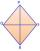Calculate the length of the two diagonals of the diamond if: a = 13 cm v = 12 cm
4. DiamondDetermine the side of diamond if its content is S = 353 cm2 and one diagonal u2 = 45 cm.
5. Rhombus 4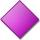Circumference of the rhombus is 44 cm, its height is 89 mm long. Calculate its content area.
6. RhombusCalculate the perimeter and area of ​​rhombus whose diagonals are 38 cm and 55 cm long.
7. SquareCalculate the perimeter and the area of square with a diagonal length 30 cm.
8. RT 10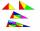Area of right triangle is 84 cm2 and one of its cathethus is a=10 cm. Calculate perimeter of the triangle ABC.
9. Rhombus HP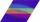Calculate area of the rhombus with height 24 dm and perimeter 12 dm.
10. Right triangle ABCCalculate the perimeter and area of a right triangle ABC, if you know the length of legs 4 cm 5.5 cm and 6.8 cm is hypotenuse.
11. RhombusCalculate the length of the diagonal AC of the rhombus ABCD, if its perimeter is 84 dm and the other diagonal BD has length 20 dm.
12. RhombusFind the length of the other diagonal and area of rhombus. The perimeter of a rhombus is 40 cm and one of the diagonals is of length 10 cm.
13. RhombusIf we increase the rhombus side by 2.4 cm; its perimeter is 20 cm. How long was the side of the rhombus?
14. Diagonals in diamons/rhombusRhombus ABCD has side length AB = 4 cm and a length of one diagonal of 6.4 cm. Calculate the length of the other diagonal.
15. RhombusThe rhombus with area 68 has one diagonal is longer by 6 than second one. Calculate the length of the diagonals and rhombus sides.
16. UmbrellaCan umbrella 75 cm long fit into a box of fruit? The box has dimensions of 390 mm and 510 mm.
17. SatinSanusha buys a piece of satin 2.4 m wide. The diagonal length of the fabric is 4m. What is the length of the piece of satin?